Science, Maths & Technology

### Become an OU studentThe evolving Universe

Start this free course now. Just create an account and sign in. Enrol and complete the course for a free statement of participation or digital badge if available.

# 1.2 Time, space, temperature and energy

The conventional view of the Universe is that, at the very instant of the Big Bang, the Universe came into being. There was no 'before' this instant since the Big Bang marked the creation of time. No location for this event can be specified since the Big Bang marked the creation of space. All that can be discussed are times after the Big Bang, and things that happen in the space created as a result of it. This is a difficult concept to visualize; but please bear with us and examine the consequences that follow.

The thread running through this course is one of a Universe in which space is forever expanding, and in which the temperature is forever falling. In the early part of its history, every time the Universe increased in age by a factor of one hundred, it also cooled by a factor of ten and distances within the Universe increased by a factor of ten.

If you are not familiar with powers of ten notation the box below will help.

## Numbers: very large and very small

When discussing the Universe, it is frequently necessary to refer to very large and very small numbers. Scientists and mathematicians have developed a short hand way of writing such numbers for convenience. The easiest way to demonstrate this is with a few examples.

(a) Large numbers

First, a few simple ones:

• 100 = 10 × 10 and is written as 102 (pronounced 'ten to the power two')

• 1000 = 10 × 10 × 10 and is written as 103 ('ten to the power three')

• 1000 000 = 10 × 10 × 10 × 10 × 10 × 10 and is written as 106 ('ten to the power six')

So the power to which the number 10 is raised (sometimes called the index or exponent) is simply the number of times 10 is multiplied by itself to make the number in question. More complicated numbers can also be written in a similar way. For instance

• 3456 = 3.456 × 10 × 10 × 10 and is written as 3.456 × 103

• 987 000 = 9.87 × 10 × 10 × 10 × 10 × 10 and is written as 9.87 × 105

The last two examples are said to be written in scientific notation: that is, as a number between 1 and 10, multiplied by 10 raised to some power. To enter such a number into your calculator will generally involve a button labelled 'E' or 'EXP' (standing for 'exponent'). So the example above would be entered as: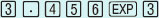. Make sure you know how to enter numbers in scientific notation into your own calculator.

Another useful way of describing large numbers is by using the following prefixes:

kilo (k) = 103; mega (M) = 106; giga (G) = 109

For instance, you will be familiar with the idea that 1000 metres (103 m) is one kilometre (1 km). Other units of measurement may also be prefixed by these terms to indicate larger quantities. Finally, we will also sometimes refer to the number 109 as a billion.

(b) Small numbers

So much for large numbers, but what about small numbers? Well, it turns out that small numbers can be treated in a similar way. Again, a few simple examples to start with:

• 0.1 = 1/10 and is written as 10−1 ('ten to the power minus one')

• 0.01 = 1/(10 × 10) and is written as 10−2 ('ten to the power minus two')

• 0.000 01 = 1/(10 × 10 × 10 × 10 × 10) and is written as 10−5 ('ten to the power minus five')

More complicated numbers can also be written in a similar way. For instance:

• 0.987 = 9.87/10 and is written as 9.87 × 10−1

• 0.000 034 56 = 3.456/(10 × 10 × 10 × 10 × 10) and is written as 3.456 × 10−5

Once again, these small numbers are written in scientific notation because they are given as a number between 1 and 10 multiplied by 10 raised to some power, in this case a negative index or exponent. Make sure you know how to enter numbers in scientific notation with negative exponents into your calculator too. If in doubt, refer to your calculator's operating manual.

There are also a few prefixes that are useful for describing very small numbers, namely:

milli (m) = 10−3; micro (μ)= 10−6; nano (n) = 10−9

Notice that the symbol for micro is the Greek letter mu, simply because the letter m is already used for the prefix milli. As an example of the use of these prefixes, you will no doubt be familiar with the fact that one-thousandth of a metre (10−3 m) is one millimetre (1 mm). Other units of measurement may also be prefixed by these terms to indicate smaller quantities.

(c) Combining large and small numbers

The use of powers of ten often makes it easier to carry out calculations too. This is because, when doing multiplication or division, the powers of ten are simply added or subtracted respectively. As usual, some examples will illustrate this. First, multiplication:

• 104 × 103 = 10(4 + 3) = 107 (i.e. 10 000 × 1000 = 10 000 000)

• 105 × 10−3 = 10(5 − 3) = 102 (i.e. 100 000 × 0.001 = 100

• 10−2 × 103 = 10(−2 + 3) = 101 (i.e. 0.01 × 1000 = 10)

and also division:

• 104/103 = 10(4 − 3) = 101 (i.e. 10 000/1000 = 10)

• 105/10−3 = 105 − (− 3) = 108 (i.e. 100 000/0.001 = 100 000 000)

## Question 1

When the Universe was 1 s old, its temperature was 1010 K. What was the temperature of the Universe when it was (a) 100 s old, and (b) 104 s old?

(a) As the Universe aged from 1 s to 100 s (a factor of one hundred increase in age), so its temperature fell by a factor of ten from 1010 K to 109 K. (b) As it aged by another factor of one hundred from 100 s to 104 s, its temperature fell by another factor of ten from 109 K to 108 K.

The fact that the separations between objects increase only by a factor of ten for every factor of one hundred increase in the age of the Universe, actually means that the expansion rate is decreasing. For instance, two objects that were (say) 105 km apart when the Universe was 1 s old would be 106 km apart when the Universe was 100 s old, and 107 km apart after 104 s.

## Worked example 1

What is the average recession speed of one of the objects referred to above, as measured from the other object, (a) when the Universe is between 1 s and 100 s old and (b) when it is between 100 s and 104 s old?

(a) You know that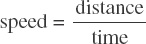and that over the interval between 1 s and 100 s, the separation increases from 105 km to 106 km. Therefore the average recession speed over this interval is given by: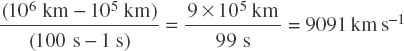This is of the order of 104 km s−1.

(b) In the interval between 100 s and 104 s, the separation increases at an average rate of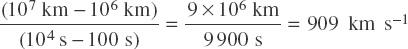which is of the order of 103 km s−1.

So even though distances between objects increase by the same factor (i.e. ten) for every hundred-fold increase in time, the expansion rate of the Universe (as given by the recession speed) actually decreases with time. The expansion rate continues to decrease at later times. This contributes to the decrease in the value of the Hubble constant with time, and is due to the gravitational attraction between all the matter in the Universe. The effect is referred to as deceleration.

The rate of cooling and expansion has changed somewhat since those early times and, as you will see later, the rate of expansion may actually have increased at some epochs. However, a consequence of the cooling and expansion is that the mean energy per particle (i.e. the energy available for any reaction to occur) is continuously reduced. This has important implications for the ways in which the four fundamental interactions manifest themselves at different epochs.

The four fundamental interactions, that is electromagnetic interaction, weak interaction, strong interaction and gravitational interaction, have very different strengths and act on different types of particle. A clue to their unification is that the strengths of the interactions vary with the energy of their environment. Furthermore, at very high energies, particles can transform into different types — quarks into leptons for instance. So the fact that only quarks feel the strong interaction whilst leptons do not, is irrelevant at very high energies.

Figure 1 shows that at higher and higher energies, first the electromagnetic and weak interactions become unified as energies reach around 1000 GeV (GeV stands for giga electronvolt - the electronvolt is a unit of energy corresponding to the energy gained by an electron in passing from one terminal of a one volt battery to the other). Then the strong interaction becomes unified with the electroweak interaction at an energy of around 1015 GeV. Finally, at the very highest energies of at least 1019 GeV, gravity too may become unified with all the other interactions.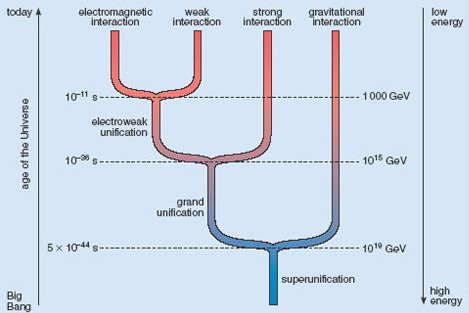Figure 1 The unification of the four fundamental interactions as energy increases towards the bottom of the diagram. As the Universe has aged, so the mean energy of each particle has fallen, and the various interactions have become distinct from one another as the energy has fallen below the thresholds shown. The approximate ages of the Universe when each of these interactions became distinct are also shown.

At the very earliest times, the Universe was extremely hot, the mean energy available per particle was extremely high, and so the unification of interactions would have occurred naturally. As the Universe has cooled, the available energy has fallen, and the interactions have in turn become distinct until the current situation is reached in which four different interactions are observed. The relationship between the mean energy of a particle and the temperature of the Universe, and the time at which such energies and temperatures applied, is shown in Figure 2.

You will appreciate from Figure 2 that the rest of this course will necessarily refer to incredibly small times after the Big Bang (notice how far along the graph 1 second appears). Many important processes took place when the Universe was significantly less than 1 s old, when the energy available for processes in the Universe was extremely high. In fact, most of the important processes were completed by the time the Universe was only a few minutes old! For each of the intervals under discussion in Sections 2 to 7 (and shown in Figure 2) the time, temperature and energy ranges are given. As you read through these sections you can imagine yourself travelling down the line of the graph in Figure 2.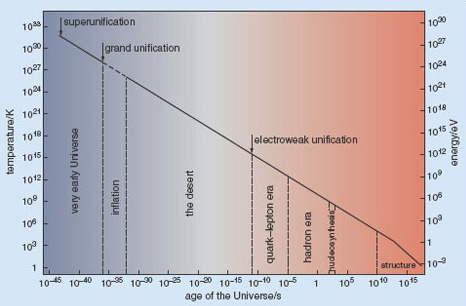Figure 2 The mean energy per particle and temperature of the Universe at different times in its history. The divisions of this graph are described in Sections 2-7. Notice that the axes are shown on powers-of-ten scales to accommodate the vast ranges of energy, temperature and time that are necessary. You will find it useful to refer back to Figure 1 and Figure 2 as each successive stage in the history of the Universe is introduced.